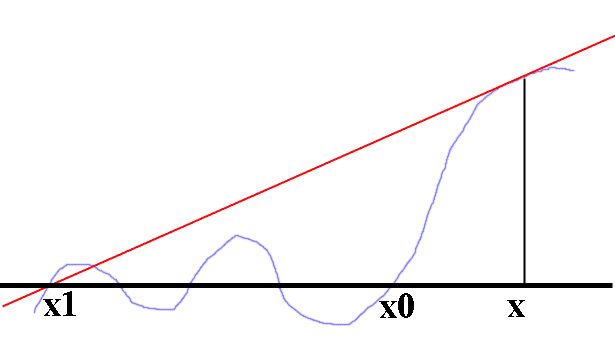# Newton's Method- finding only positive zeros

• erkokite

#### erkokite

I have a situation where I am using Newton's Method to solve a highly nonlinear, non-analytic equation with both positive and negative zeros. My situation requires that only positive zeros be found. Does anyone know any extensions to NM to restrict the solutions to greater than zero?

Thanks.

Suppose you were to chose a starting point greater than any +ve root?

As far as I know there is no method that can guarantee anything.

I agree with both comments already posted. Pure Newton's method, if it finds a solution (not guaranteed to find one), will find the one closest to the starting point, which isn't necessarily the desired one.

Having said that, there are things you can potentially do, depending on the details of the specific problem you have. One is to try several different starting points and hope that you'll eventually find one that produces the result you want. If the problem has only 1 variable, it should be straightforward to plot f(x) vs x and graphically (or analytically) find an approximate root that you want, and use it as a starting point in Newton's Method.

I believe there is a fair body of publication material on the general topic of optimization with inequality constraints (i.e. minimize f(x) where x >= 0). There might be similar info on finding zeros of functions, I really don't know much about them.

A couple of methods I'm aware of are based on a user defined range for the solution. One is Brent's Method:

http://en.wikipedia.org/wiki/Brent's_method

Another is a random method, where f(x) is calculated for randomly generated values for x (within user specified limits), ranked according to value of f(x), a new range defined from the ranking, then repeat.

In both cases, the result could be used as a starter for Newton's Method, but does require you to specify an upper bound on the solution (lower bound would be zero), which may not be straightfoward.

Last edited:
Pure Newton's method, if it finds a solution (not guaranteed to find one), will find the one closest to the starting point

I don't think so.Starting point x, yet solution found is x1, not x0. You need additional conditions for the statement to be true.

I don't think so.

Ah, right you are. I stand corrected.

You're welcomeTo elaborate on Borek's comment, you can take additional steps to increase the odds of finding the root nearest to the starting point. One option is to take partial Newton steps, that is, use xi+1 = xi - mu*f/f', where mu is between 0 and 1. The smaller mu is, the more steps are needed to converge, but the less likely to miss the nearest root.

Another option would be to use a higher order method such as Halley's method that utilizes the 2nd derivative as well as the first derivative.

http://en.wikipedia.org/wiki/Halley's_method

#### Attachments

Last edited:
It is usual to roughly locate the roots, eg by sign change and perhaps bisection, before embarking on any refinement method, such as Newton's.

You haven't told us about your function or even if it is continuous as you require derivatives for N.

If you can get close enough to the root to start with real world functions will, in general, converge.
So if the distance between the greatest root and X0 is halved N would indeed converge to it.

I am aware that it is always possible to construct counter examples, but you should stake out your territory beforehand.